Courses

## 16 Questions MCQ Test Chemistry Class 11 | Test: Electromagnetic Radiations

Description
This mock test of Test: Electromagnetic Radiations for Class 11 helps you for every Class 11 entrance exam. This contains 16 Multiple Choice Questions for Class 11 Test: Electromagnetic Radiations (mcq) to study with solutions a complete question bank. The solved questions answers in this Test: Electromagnetic Radiations quiz give you a good mix of easy questions and tough questions. Class 11 students definitely take this Test: Electromagnetic Radiations exercise for a better result in the exam. You can find other Test: Electromagnetic Radiations extra questions, long questions & short questions for Class 11 on EduRev as well by searching above.
QUESTION: 1

### Direction (Q. Nos. 1-8) This section contains 8 multiple choice questions. Each question has four choices (a), (b), (c) and (d), out of which ONLY ONE option is correct.   Q. FM radio broadcasts at 900 kHz. What wavelength does this corresponds to?

Solution: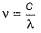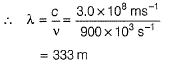QUESTION: 2

### The nucleus of helium contains

Solution:

A helium nucleus is made of two protons and two neutrons.

They both have strong interactions.

A helium nucleus has a charge +2.

QUESTION: 3

### Moseley’s equation is represented as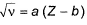where, a and b are constants.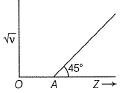Q. If OA = 1, then atomic number of the element showing frequency of 400 Hz is

Solution: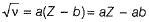Thus, graph is a straight line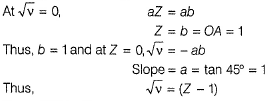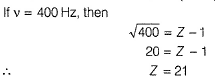QUESTION: 4

A gas absorbs photon of wavelength 355 nm and emits at two wavelengths. If one of the emissions is at 680 nm, the other is at

[AlEEE 2011]

Solution: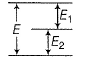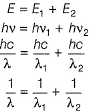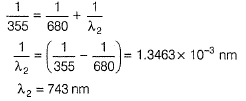QUESTION: 5

The longest wavelength of light capable of breaking a single (Cl — Cl) bond in Cl2 is if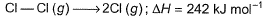[AlEEE 2010]

Solution:

ΔH = 242 kJ mol-1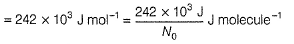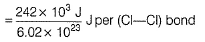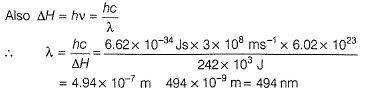QUESTION: 6

Which of the following conclusions could not be derived from Rutherford’s α-particle scattering experiment?

Solution:

(a) Electrons (-) and nucleus (+) are held togeather by electrostatic forces of attractions. Thus. true.
(b) Radius of the atom (= 10-10m) > radiue of the nucleus (10-15m). Thus true
(c) Most of the space is empty as given by radius of the nucleus and atom. Thus, true.
(d) As electron moves in a circular paith, it emits energy and ultimately false into nucleus. Thus, false.

QUESTION: 7

Which of the following statements is not correct about the characteristics of cathode rays?

Solution:

(d) Cathode rays consist of negatively charged material particles called electron. They were discovered by William Crookes. The characteristics of cathode rays do not depend upon the material of electrodes and the nature of the gas present in the cathode ray tube.

QUESTION: 8

Energy of a mole of radio wave photons with a frequency of 909 kHz is

Solution:

E = N0hv

= 6.02 x 1023 x 6.62 x 10-34 Js x 909 x103 s-1
= 3.62 x 10-4 J

*Multiple options can be correct
QUESTION: 9

Direction (Q. Nos. 9-11) This section contains 3 multiple choice questions. Each question has four choices (a), (b), (c) and (d), out of which ONE or  MORE THANT ONE  is correct.

Q. Select the correct statement(s).

Solution:

(a) Oscillations travel through space at a velocity of light, thus electromagnetic radiation is in the form of energy E = hv-true.
(b) Radiowaves, microwaves, X-rays are part of visible light -thus, true.
(c) Some of the radiations are absorbed in the atmosphere, hence spectrum differ - true.
(d) Ozone layer absorbs ultraviolet radiation - true.

*Multiple options can be correct
QUESTION: 10

Consider the following transitions n = 1 to n = 2 and then, n = 2 to n = 3.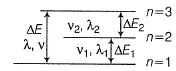Then

Solution: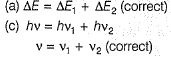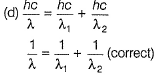Thus, (b) is incorrect.

*Multiple options can be correct
QUESTION: 11

Select the correct statement(s).

Solution:

(a) Correct
(b) As temperature increases, distribution of frequency (and energy) increases. Thus, correct.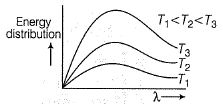(c) As temperature increases, radiation emitted is shifted to lower wavelength, i.e. higher frequency. Correct
(d) All types of radiations have same speed 3 x 108 ms-1. Correct

QUESTION: 12

Direction (Q. Nos. 12 and 13) This section contains a paragraph, wach describing  theory, experiments, data etc. three Questions related to paragraph have been  given.Each question have only one correct answer among the four given  ptions  (a),(b),(c),(d)

A hypothetical electromagnetic wave is pictured here.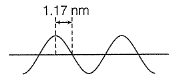Q. Energy associated with this wave is

Solution:

A to E makes one complete wave.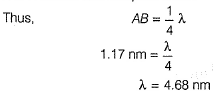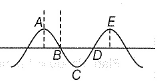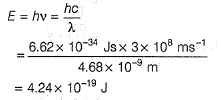If there are n nodes including those at the ends, in a string of length /, then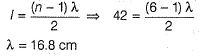QUESTION: 13

A hypothetical electromagnetic wave is pictured here.Q. A standing wave in a string 42 cm long has a total of six nodes (including those at the ends). Wavelength of the standing wave is

Solution:

A to E makes one complete wave.If there are n nodes including those at the ends, in a string of length /, thenQUESTION: 14

Direction (Q. Nos. 14) Choice the correct combination of elements and column I and coloumn II are given as option (a), (b), (c) and (d), out of which ONE option is correct.

Q. The colours of visible light are at different wavelength. Match the wavelengths in Column I with their colours in Column II.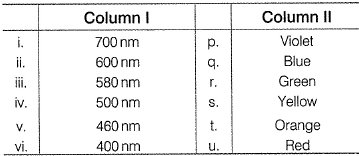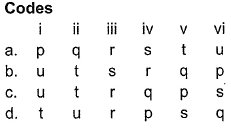Solution: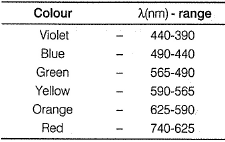Thus, (i)(700nm) Red (u)
(ii) (600 nm) - Orange (t)
(iii) (580 nm) - Yellow (s)
(iv) (500nm) - Green (r)
(v) (460 nm) - Blue (q)
(vi) (400 nm) - Violet (p)

*Answer can only contain numeric values
QUESTION: 15

Direction (Q. Nos. 15 and 16) This section contains 2 questions. when worked out will result in an integer from 0 to 9 (both inclusive)

Q.

In Millikan’s experiment, static electric charge on the oil drops has been obtained by shining X-rays. If the static electric charge on the oil drop is -1.282x 10-18 C, calculate the number of electrons present on it.

Solution:

Charge on one electron = - 1.6022 x 10-19C
Let, number of electrons = N
Then total charge = - N x 1.6022 x 10-19C
Given, total charge = -1.282 x 10-18 C
Thus, - N x 1.6022 x 10-19 C = - 1. 282 x 10-18C

∴ N = 8

*Answer can only contain numeric values
QUESTION: 16

Energy change associated per mole of atoms with an atomic transition giving rise to radiations of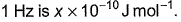What is the value of x?

Solution:

Energy = n.hv where n = 1 mole or 6.023 × 1023 So E = 6.023 ×1023 × 6.626×10-34 × 1 = 3.91 × 10-10 so approximately value of x = 4.